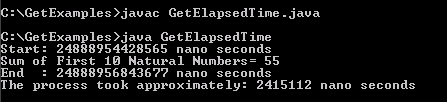# Java get Elapsed Time

In this section, you will study how to obtain the elapsed time. As you all know that the Elapsed time is the time taken to complete the process.### Java get Elapsed Time

In this section, you will study how to obtain the elapsed time. As you all know that the Elapsed time is the time taken to complete the process.# Java get Elapsed Time

In this section, you will study how to obtain the elapsed time. As you all know that the Elapsed time is the time taken to complete the process. In the given example, we have used the method System.nanoTime() to get the start time and the end time of the defined process. Here we are calculating the sum of first ten natural numbers in order to find the elapsed time by subtracting the start time from the end time of the process.

Here is the code of GetElapsedTime.java

 ``` public class GetElapsedTime {   public static void main(String[] args) {   long start = System.nanoTime();   System.out.println("Start: " + start+" nano seconds");     int sum = 0;   for (int i = 0; i <= 10; i++) {   sum = sum + i;   }   System.out.println("Sum of First 10 Natural Numbers= "+sum);   long end = System.nanoTime();   System.out.println("End  : " + end+" nano seconds");     long elapsedTime = end - start;   System.out.println("The process took approximately: "   + elapsedTime + " nano seconds");   } }```

Output will be displayed as: